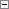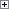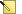A repetitive statement is also known as a loop. The following table shows the looping mechanisms in C/AL.

Looping mechanism Description

FOR

Repeats the inner statement until a counter variable equals the maximum or minimum value specified.

WHILE

Repeats the inner statement as long as the specified condition is TRUE. The statement in a loop of this kind is repeated 0 or more times.

REPEAT

Repeats the inner statements until the specified conditions evaluate to TRUE. The statements in a loop of this kind are always executed at least one time.

FOR-TO and FOR-DOWNTO Control Structure

The following syntax shows the FOR-TO and FOR-DOWNTO statement.Copy Code
FOR <Control Variable> := <Start Number> TO <End Number> DO
<Statement>

The data type of <Control Variable>, <Start Number>, and <End Number> must be Boolean, number, time, or date.

Use FOR statements when you want to execute code a specific number of times.

Use a control variable to control the number of times that the code is executed. The control variable can be increased or decreased by one, depending on whether TO or DOWNTO is used.Note
When the FOR statement is executed, <Start Number> and <End Number> are converted to the same data type as <Control Variable> if it is required. This type conversion can cause a run-time error.

When using a FOR-TO loop, the <Statement> will not be executed if the <Start Number> is greater than the end value. When using a FOR-DOWNTO loop, the <Statement> will not be executed if the <Start Number> is less than the end value.Note
If the value of the control variable is changed inside the FOR loop, then the behavior is not predictable. Furthermore, the value of the control variable is undefined outside the scope of the FOR loop.

Example 1

Create the following variable.

Variable Data type

Count

Integer

The following code initiates a FOR loop that uses the integer control variable named Count.Copy Code
FOR Count := 1000 TO 100000000000000 DO

When this statement is executed, a run-time error occurs because the start and end values are converted to the same data type as the Count control variable. Count has been declared as an integer variable. The end number 100000000000000 is outside the valid range for integers, and an error occurs.

Example 2

The following example shows how to nest FOR statements.

Create the following variables.

Variable Data type

I

Integer

J

Integer

Set the Dimensions property of variable A to 5;7.

The following FOR statements could be used to initialize every element in a 5x7 array with the value 23.Copy Code
FOR I := 1 TO 5 DO
FOR J := 1 TO 7 DO
A[I,J] := 23;

WHILE-DO Control Structure

The following syntax shows the WHILE-DO statement.Copy Code
WHILE <Condition> DO
<Statement>

If <Condition> is TRUE, then <Statement> is executed repeatedly until <Condition> becomes FALSE. If <Condition> is FALSE from the start, then <Statement> is never executed.

The WHILE DO statement can be used when some code should be repeated as long as an expression is TRUE.

Programming Conventions

When there is only one condition, put WHILE and DO on the same line. Put the statements on separate lines and indented by two spaces.

When there are multiple conditions, put the conditions on separate lines and indented by two spaces and put DO on a separate line that is aligned with WHILE.

ExampleCopy Code
WHILE <expr> DO
<Statement>;

ExampleCopy Code
WHILE <expr> DO BEGIN
<Statement>;
<Statement>;
END;

ExampleCopy Code
WHILE <expr> AND
<expr> AND
<expr>
DO BEGIN
<Statement>;
<Statement>;
END;

Example

Create the following variable.

Variable Data type

I

Integer

The following C/AL code increases the variable I until it equals 1000 and displays a message when it is finished.Copy Code
WHILE I < 1000 DO
I := I + 1;
Message(format(I));

REPEAT-UNTIL Control Structure

The following syntax shows the REPEAT-UNTIL statement.Copy Code
REPEAT
<Statements> UNTIL <Condition>

<Statements> is executed repeatedly until <Condition> is TRUE.

The REPEAT UNTIL control structure resembles the WHILE control structure. The difference is that because the REPEAT UNTIL statement is executed from left to right, the <Statements> is always executed at least one time, regardless of what the <Condition> is evaluated to. This contrasts with the WHILE control structure, which performs the evaluation before the <Statement> is executed. In the WHILE control structure, if the first evaluation of <Condition> returns FALSE, then no statements are executed.

Programming Conventions

Always put REPEAT on a separate line.

ExampleCopy Code
IF x < y THEN BEGIN
REPEAT
x := x + 1;
a := a - 1;
UNTIL x = y;
b := x;
END;

Example

Create the following variables.

Variable Data type Subtype

Count

Integer

Customer

Record

CustomerCopy Code
Count := 0;
IF Customer.FIND('-') THEN
REPEAT
Count := Count + 1;
UNTIL Customer.NEXT <= 0;
Message(’The Customer table contains %1 records.’,Count);

This code uses a REPEAT-UNTIL loop to count the number of entries in the Customer table. The FIND function finds the first entry in the table. Each time NEXT is called, it steps one record forward. When NEXT equals 0, there are no more entries in the table. The loop is exited, and a message displays how many entries were found.

EXIT Statement

The EXIT statement is used to control the flow of the execution. The following syntax shows an EXIT statement.Copy Code
EXIT([<Value>])

An EXIT statement is used to interrupt the execution of a C/AL trigger. The interruption occurs even when the code is executed inside a loop or a similar structure. The EXIT statement is also used when a local function should return a value.

Using EXIT without a parameter in a local function corresponds to using the parameter value 0. The C/AL function will return the value 0 or '' (empty string).

A compile-time error occurs if EXIT is called by using a return parameter from either of the following:

• System-defined triggers.
• Local functions that do not return a value.

Example

The following example shows the use of the EXIT statement in a local function. Assume that the IF statement is used to detect an error. If the error condition is met, then execution is stopped and the local function returns the error code 1.Copy Code
FOR I := 1 TO 1000 DO BEGIN
IF Amount[I] < Total[I] THEN
EXIT(1);
A[I] := Amount[I] + Total[I];
END;